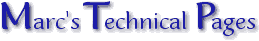Cheating withAntenna Gain In a recent email it was asked: "I am looking into buying an antenna for my boat and am trying to figure out what antenna to buy. Which antenna will have a better range - a 3 foot with 6db gain or a 5 foot with a 3db gain?" There are two ways one could answer this question, the short way being "the longer one", or with a full explanation as done below. Here's hoping you appreciate the full answer! Before we start it is being assumed that the international boating VHF frequency band around 156MHz is being referred to (just in case you wonder where the frequency suddenly crept in). Ahead of stripping the antennas to shreds, let's first create a suitable baseline from which to work. Wavelength is critical with all antennas. Contrary to popular belief length is gain, or conversely to get gain one needs length. There is a 'reference' by which all reputable companies work being the half wavelength dipole. Wavelength is a simple calculation of 300/MHz = metres. Thus for 156 MHz the wavelength is 1.92m making a ½-wavelength 0.96m or 3.15ft (about as long as your 6dB one). The smallest omni-directional gain antenna is 5-eighths wavelength or 4ft. Above that you start getting strange beasts called 'collinears', the most basic model being '½ over ¼' thus 5ft. Now for gain. As said, reputable companies express their gain with reference to a ½-wavelength dipole (this being assumed to have no gain and therefor = 0dB). Cheating companies reference against a theoretical antenna known as an 'isotropic' antenna which is effectively a single point radiating spherically in all directions. Such an antenna does not exist but can be mathematically calculated and the difference between it and a half wave is 2.14dB. As the the ½-wave has a radiation pattern the points of maximum radiation show a 'gain' of the said 2.14dB (see "Antenna Gain Explained" for radiation patterns). Let's start with the smaller antenna. If 3ft is a half wavelength and this is the reference of 0dB how can the manufacturer quote 6dB gain!? Simple, they are not quoting true gain but 'realised' gain. This is as a result of the radiation pattern of a ¼-wave (which is at 45°) being 'measured' against that of the ½-wave (which is 0°) creating a sense of extra power 'realised' along the desired path which is horizontal. This realised gain is about 3dB, added to the 2.14dB 'gain' over an isotropic and then rounded off gives one about 6dB. Now the long antenna (5ft). Here it is believed the antenna to be more in line with the truth. It sounds like a 5-eighths (groundplane required) or a J-pole (no groundplane required). If the former then there is a gain of 1.85dB, which if added to the basic 2.14dB isotropic-dipole difference, gives you about 4dBi. Radio guys like to work in "doubling of power" figures i.e. 3dB so this is probably where this came from. From the article "Choosing the correct antenna" we can see the 5-eighths is a very preferred antenna for "mobile" work. We're just talking about a "mobile on water".| | Ask a Question | © 14.03.01